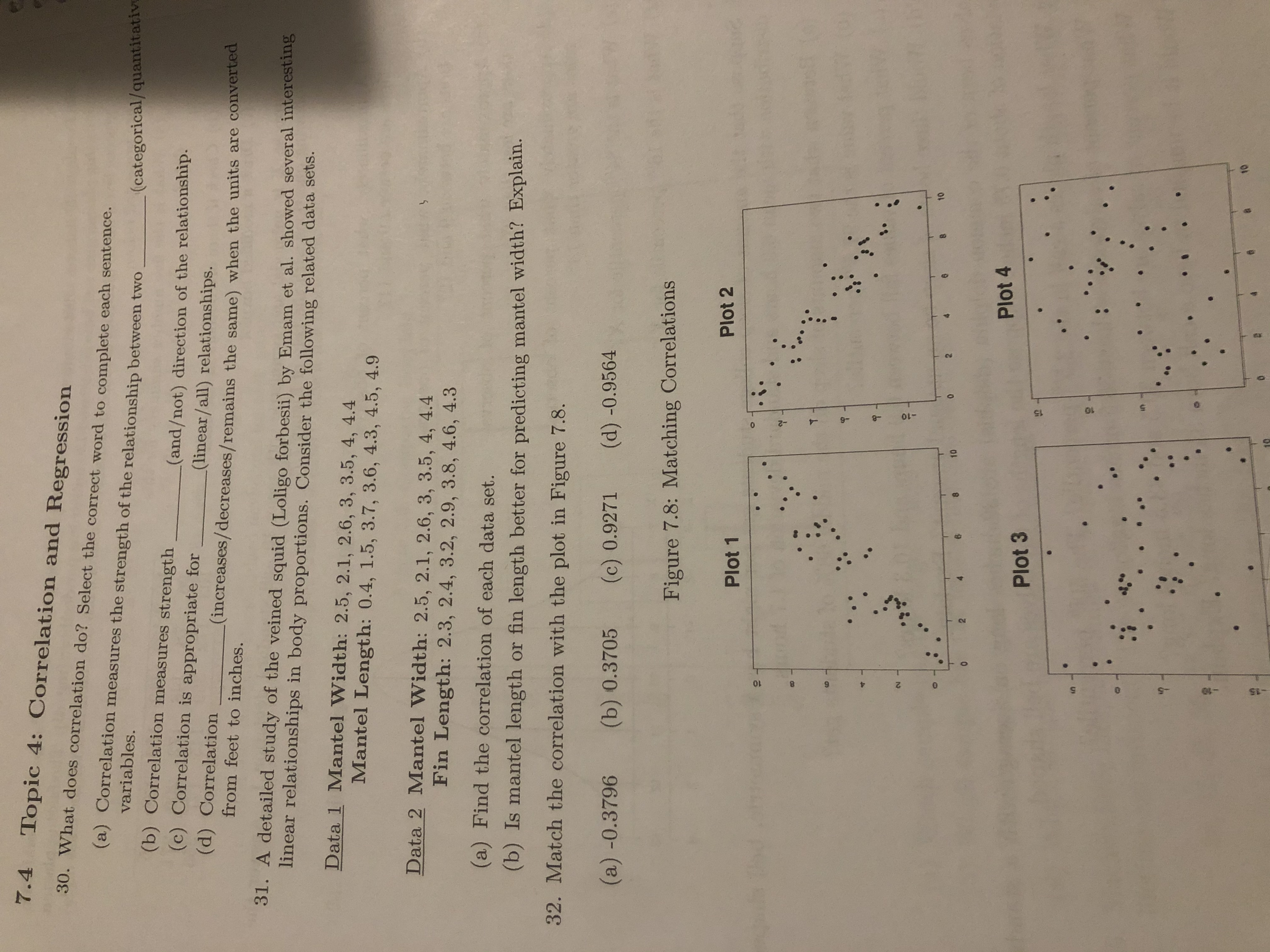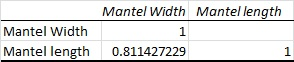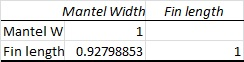# 7.4 Topic 4: Correlation and Regression30. What does correlation do? Select the correct word to complete each sentence.(a) Correlation measures the strength of the relationship between twovariables.(categorical/quantitative(b) Correlation measures strength(and/not) direction of the relationship.(linear/all) relationships.(increases/decreases/remains the same) when the units are converted(c) Correlation is appropriate for(d) Correlationfrom feet to inches.31. A detailed study of the veined squid (Loligo forbesii) by Emam et al. showed several interestinglinear relationships in body proportions. Consider the following related data sets.Data 1 Mantel Width: 2.5, 2.1, 2.6, 3, 3.5, 4, 4.4Mantel Length: 0.4, 1.5, 3.7, 3.6, 4.3, 4.5, 4.9Data 2 Mantel Width: 2.5, 2.1, 2.6, 3, 3.5, 4, 4.4Fin Length: 2.3, 2.4, 3.2, 2.9, 3.8, 4.6, 4.3(a) Find the correlation of each data set.(b) Is mantel length or fin length better for predicting mantel width? Explain.32. Match the correlation with the plot in Figure 7.8.(a) -0.3796(b) 0.3705(d) -0.9564(c) 0.9271Figure 7.8: Matching CorrelationsPlot 2Plot 1102.10Plot 4Plot 3104.8.-8-2

Question
343 views

Question 31 parts A and Bhelp_outlineImage Transcriptionclose7.4 Topic 4: Correlation and Regression 30. What does correlation do? Select the correct word to complete each sentence. (a) Correlation measures the strength of the relationship between two variables. (categorical/quantitative (b) Correlation measures strength (and/not) direction of the relationship. (linear/all) relationships. (increases/decreases/remains the same) when the units are converted (c) Correlation is appropriate for (d) Correlation from feet to inches. 31. A detailed study of the veined squid (Loligo forbesii) by Emam et al. showed several interesting linear relationships in body proportions. Consider the following related data sets. Data 1 Mantel Width: 2.5, 2.1, 2.6, 3, 3.5, 4, 4.4 Mantel Length: 0.4, 1.5, 3.7, 3.6, 4.3, 4.5, 4.9 Data 2 Mantel Width: 2.5, 2.1, 2.6, 3, 3.5, 4, 4.4 Fin Length: 2.3, 2.4, 3.2, 2.9, 3.8, 4.6, 4.3 (a) Find the correlation of each data set. (b) Is mantel length or fin length better for predicting mantel width? Explain. 32. Match the correlation with the plot in Figure 7.8. (a) -0.3796 (b) 0.3705 (d) -0.9564 (c) 0.9271 Figure 7.8: Matching Correlations Plot 2 Plot 1 10 2. 10 Plot 4 Plot 3 10 4. 8. -8 -2 fullscreen
check_circle

Step 1

a).

The correlation between Mantel Width and Mantel length is as follows:

Step-by-step procedure to obtain the correlation using Excel is as follows:

• First enter the data set into Excel spread sheet.
• Go to data option in ribbon pan.
• Select data analysis.
• Click on Correlation and select the input range.
• Click Ok.
Step 2

Output obtained using Excel is represented as follows:Thus, the correlation between Mantel Width and Mantel length is 0.8114.

Step 3

The correlation between Mantel Width and Fin length is as follows:

Step-by-step procedure to obtain the correlation using Excel is as follows:

• First enter the data set into Excel spread sheet.
• Go to data option in ribbon pan.
• Select data analysis.
• Click on Correlation and select the input range.
• Click ok.

Output obtained using Excel is as follows:Fro...

### Want to see the full answer?

See Solution

#### Want to see this answer and more?

Solutions are written by subject experts who are available 24/7. Questions are typically answered within 1 hour.*

See Solution
*Response times may vary by subject and question.
Tagged in